Elite indicators :) - page 166118

Qqe mtf

Mladen, thanks so much for all your great work.

I cant seem to find YOUR version of an mtf QQE indicator (with the interpolating and done correctly) - if you have done one can you point me to the post please, and if not, could you possibly do one? Thanks so much!

Odysseus30

Triangular MA

Kindly consider this request. Thanks

umeshkathuria:

Attached is the TriangularMA centered abands alerts indicator.

This indicator gives alerts and emails when the price crosses the bands.

Can you modify this indicator to give alerts when:

The previous candle has touched the band and the present candle is of opposite color (Black for upper band and white for lower band).

Thanks

Umesh
umeshkathuria:

By color of candle I meant:

When the 1st candle touches the upper band it is of white color(i.e. its close is higher than its open) and 2nd candle is of black color (i.e. its close is lower than its open) then the indicator gives a Down alert.

When the 1st candle touches the Lower band it is of Black color(i.e. its close is lower than its open) and 2nd candle is of White color (i.e. its close is higher than its open) then the indicator gives an UP alert.

Its a two candle pattern with Triangular MA abands alerts

Triangular moving average is confirming the overbought/oversold condition and candle pattern is confirming the reversal.

I am using H1 timeframe for this setup.

Please find the attached image for details.

Thanks and Regards

Umesh
Files:89

I had posted earlier concerning a Dynamic Balance Point Indicator.

Apparently it's based on Drummond Geometry, the work of Robert Krause, and Chaos Theory from what I've read so far.

https://www.mql5.com/en/forum/general

mrtools found something that looks promising called SOKOL. Would it be possible to check the code to see if the parameters can be changed for the DBP calculation?

The SOKOL is coded this way

datetime today = TimeLocal (), serverTime = TimeCurrent ();

int offset = 1, currentDay = TimeDayOfWeek (today), serverDay = TimeDayOfWeek (serverTime);

if (currentDay == 0 ||

((currentDay == 6 || currentDay == 1) && serverDay == 5))

offset = 0;

double high = iHigh (0, PERIOD_D1, offset),

low = iLow (0, PERIOD_D1, offset),

close = iClose (0, PERIOD_D1, offset);

BalancePoint = (high + low + close) / 3;

and the metaquotes code settings I found are written this way,

dt:=DayOfWeek();

DBC:=(HighestSince(5,DayOfWeek()=dt,H)+

LowestSince(5,DayOfWeek()=dt,L)+CLOSE)/3;

DBC

I tried to work on the code in metaeditor and realized I have no idea what I'm doing.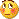I'm still a beginner with coding so this is way over my head. If you could help me with this I would really appreciate it.

Kind regards,

Fudo

I think this could be a very useful tool if it were set up properly, basing the calculation on a floating 5 day timeframe will give us a completely different vantage point of current support and resistance than the current static weekly/daily pivot methodolgy. Ultimately a MTF version with both the fixed and dynamic levels projected would be excellent for calculating higher TF support and resistance for the lower TFs.

What do you think?

Files:
sokol_1.mq4 11 kb151205

Fudo

From the description this is one version (it is a daily dynamic balance point on a 4 hour chart - I post this as an example even if it is not supposed to work on a lower time frame, but that is a deviation that I decided to make):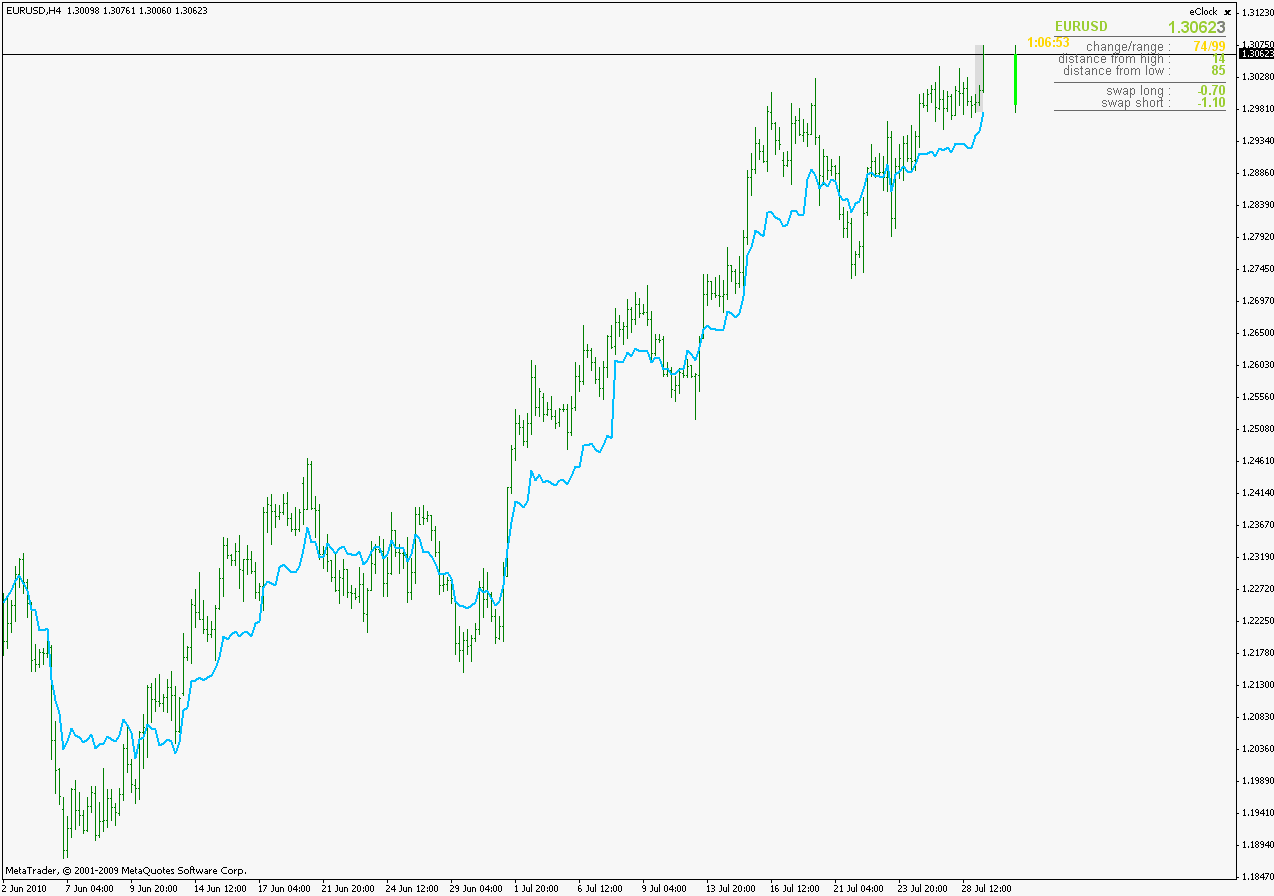_____________________________ Some explanations :
Even if it uses time frame as parameter it is not a multi time frame indicator. Instead, it uses that time frame to find highs and lows that are used in calculation. For example :
For a length 5 days dynamic balance point, on Friday it will use data from Monday 00:00 till the bar it calculates to find high / low and combined with current close it calculates the balance point
Parameters:

dbpLength-> length (in target time frames) to calculate

dbpTimeFrameForHighLow-> target time frame to use for calculations

_____________________________

As of Drummond geometry, the chaos and the rest that guy is talking about : forget about it. For a daily target time frame dynamic balance point is simply (highest in the last n days + lowest in the last n days + current close) / 3. The metastock code is a weekly dynamic, but I decided to make daily the default time frame (I think it is more suitable for forex) but you can easily use any time frame you like

PS: will make a version that does exactly (as the time is concerned) as the metastock version, but I think that, value wise, difference is not going to be significant

regards

Fudomyo:

I had posted earlier concerning a Dynamic Balance Point Indicator.

Apparently it's based on Drummond Geometry, the work of Robert Krause, and Chaos Theory from what I've read so far.

https://www.mql5.com/en/forum/general

mrtools found something that looks promising called SOKOL. Would it be possible to check the code to see if the parameters can be changed for the DBP calculation?

The SOKOL is coded this way

datetime today = TimeLocal (), serverTime = TimeCurrent ();

int offset = 1, currentDay = TimeDayOfWeek (today), serverDay = TimeDayOfWeek (serverTime);

if (currentDay == 0 ||

((currentDay == 6 || currentDay == 1) && serverDay == 5))

offset = 0;

double high = iHigh (0, PERIOD_D1, offset),

low = iLow (0, PERIOD_D1, offset),

close = iClose (0, PERIOD_D1, offset);

BalancePoint = (high + low + close) / 3;

and the metaquotes code settings I found are written this way,

dt:=DayOfWeek();

DBC:=(HighestSince(5,DayOfWeek()=dt,H)+

LowestSince(5,DayOfWeek()=dt,L)+CLOSE)/3;

DBC

I tried to work on the code in metaeditor and realized I have no idea what I'm doing.I'm still a beginner with coding so this is way over my head. If you could help me with this I would really appreciate it.

Kind regards,

Fudo

I think this could be a very useful tool if it were set up properly, basing the calculation on a floating 5 day timeframe will give us a completely different vantage point of current support and resistance than the current static weekly/daily pivot methodolgy. Ultimately a MTF version with both the fixed and dynamic levels projected would be excellent for calculating higher TF support and resistance for the lower TFs.

What do you think?
Files:89

Wow! That was amazingly fast. Thank you so much.

That was a great idea to add the flexibility of adjusting the dbpLength and target time frame. very nice.Is there a way to have the indicator draw the balance point as a horizontal line and factor support and resistance levels off of it based on these calculations?

Resistance1 = 2 * BalancePoint - low;

Resistance2 = BalancePoint + (high - low);

Resistance3 = high + 2 * (BalancePoint - low);

Support1 = 2 * BalancePoint - high;

Support2 = BalancePoint - (high - low);

Support3 = low - 2 * (high - BalancePoint);

Fudo From the description this is what I made (it is a daily dynamic balance point on a 4 hour chart - I post this as an example even if it is not supposed to work on a lower time frame, but that is a deviation that I decided to make):_____________________________ Some explanations :
Even if it uses time frame as parameter it is not a multi time frame indicator. Instead, it uses that time frame to find highs and lows that are used in calculation. For example :
For a length 5 days dynamic balance point, on Friday it will use data from Monday 00:00 till the bar it calculates to find high / low and combined with current close it calculates the balance point
Parameters:

dbpLength-> length (in target time frames) to calculate

dbpTimeFrameForHighLow-> target time frame to use for calculations

_____________________________

As of Drummond geometry, the chaos and the rest that guy is talking about : forget about it. For a daily target time frame dynamic balance point is simply (highest in the last n days + lowest in the last n days + current close) / 3. The metastock code is a weekly dynamic, but I decided to make daily the default time frame (I think it is more suitable for forex) but you can easily use any time frame you like

regards151205

Fudo,

Will do

As of the comparison : I was rightHere is a weekly balance point (works exactly as the metastock formula - for example on the picture it is a balance point from 5 Thursdays ago till today) compared to 25 day daily balance point. Red one is the daily, blue one is the weekly.

As you can see, differences are hardly significant and they are coming from a logical error in metastock indicator : when they are calculating 5 weeks they are actually calculating 5 weeks + 1 day (today) If you set a number of days to 26 in "our" (metatrader version) you are going to get exactly the same values (see the lower picture : the thin black line enclosed within the blue line is the 26 day pboIf today is Thursday then the starting day for a 5 week period can not be Thursday but must be Friday (that is the extra day they are having)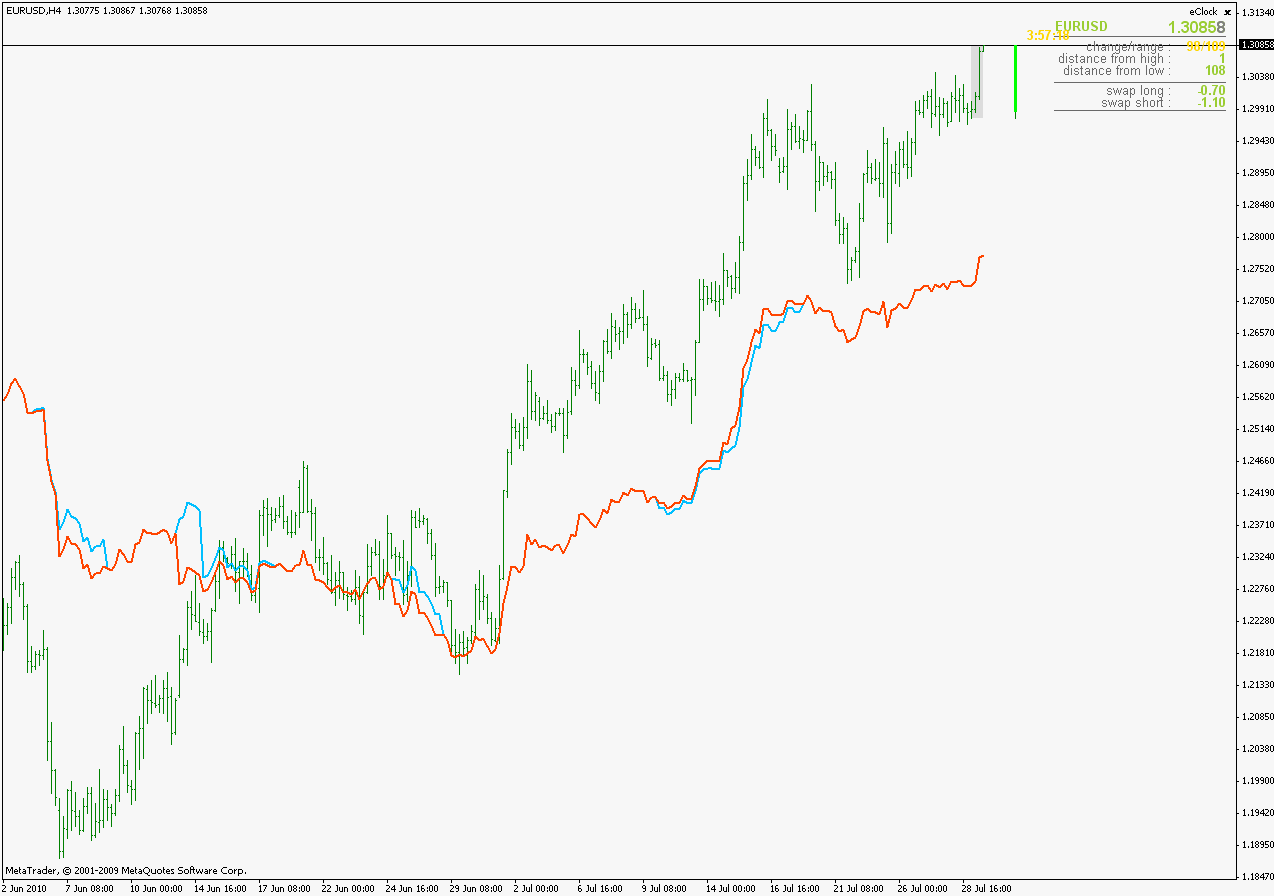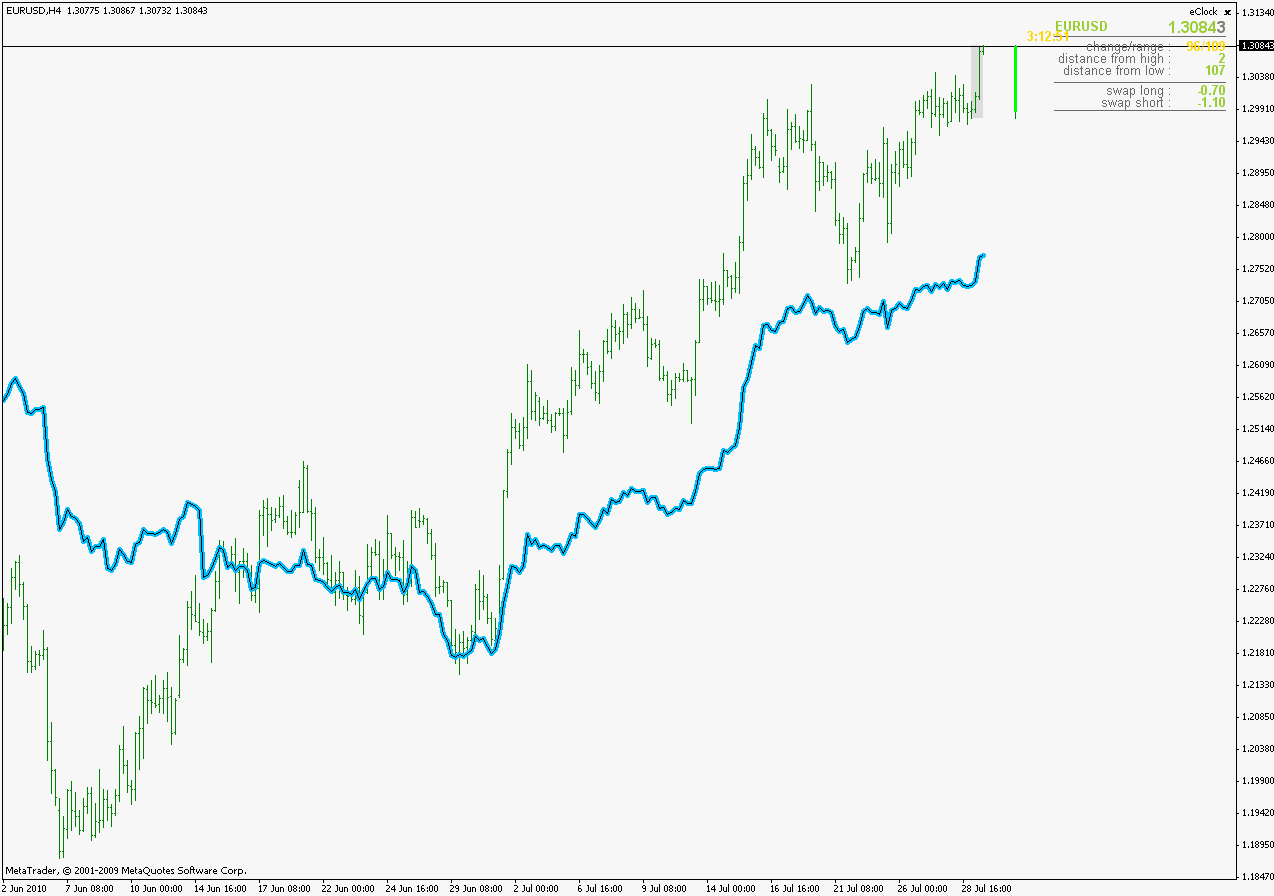regards

Files:89

You are always right.It's quite brilliant that you can visualize these things before you build them.

My mind works very differently. I get a hunch about something then need to eliminate my errors until the only thing that's left is the one that works....lol

In the spirit of muddling through, I realized the SR levels shouldn't be based on the Dynamic Balance Point.What the author is trying to acheive with this formulation is using the dynamic balanced point and the fixed balance point in tangent for trend determination.

If the DBP is above the FBP the trend is up and vice versa.

Because the dynamic balance point is the faster moving line, it wouldn't work to base SR levels off of it. SR should be based on the fixed balance point equation.

Fixed Balance Point Equation

{Fixed Balance Point Calculation}

FBC:=If(Mc1=0 AND Fc1>2,

{then}(Ref(HHV(H,LastValue(mc1)),-1)+

Ref(LLV(L,LastValue(Mc1)),-1)+

Ref(C,-1))/3,

{else}If(Fc1=0 AND Mc1>5,

{then}(HHV(H,LastValue(Fc2))+

LLV(L,LastValue(Fc2))+C)/3,

{else}If(Fc1=0,

{then}(HHV(H,LastValue(Mc1))+

LLV(L,LastValue(Mc1))+C)/3,

{else}0)));

{Fixed Balance Point Plot}

FBP:=ValueWhen(1,FBC>0,FBC);

FBP;

What's interesting is he's basing his SR levels off of this Fibonacci formulation rather than the calculation used to factor standard pivots.

(I'm not sure which would be more accurate, the fib levels or standard pivot levels)

Weekly Price Range Calculation

WRC:=If(Mc1=0 AND Fc1>2,

{then}Ref(HHV(H,LastValue(mc1)),-1)-

Ref(LLV(L,LastValue(Mc1)),-1),

{else}If(Fc1=0 AND Mc1>5,

{then}HHV(H,LastValue(Fc2))-

LLV(L,LastValue(Fc2)),

{else}If(Fc1=0,

{then}HHV(H,LastValue(Mc1))-

LLV(L,LastValue(Mc1)),

{else}0)));

WRP:=ValueWhen(1,WRC>0,WRC);

{Resistance Range}

RR1:= FmlVar("FT-FBP","FBP")+(WRP*.5);

RR2:= FmlVar("FT-FBP","FBP")+(WRP*.618);

{Support Range}

SR1:= FmlVar("FT-FBP","FBP")-(WRP*.5);

SR2:= FmlVar("FT-FBP","FBP")-(WRP*.618);

{Plot Ranges}

RR1;

RR2;

SR1;

SR2;

Fudo,

Will do

As of the comparison : I was rightHere is a weekly balance point (works exactly as the metastock formula - for example on the picture it is a balance point from 5 Thursdays ago till today) compared to 25 day daily balance point. Red one is the daily, blue one is the weekly.

As you can see, differences are hardly significant and they are coming from a logical error in metastock indicator : when they are calculating 5 weeks they are actually calculating 5 weeks + 1 day (today) If you set a number of days to 26 in "our" (metatrader version) you are going to get exactly the same values (see the lower picture : the thin black line enclosed within the blue line is the 26 day pboIf today is Thursday then the starting day for a 5 week period can not be Thursday but must be Friday (that is the extra day they are having)46

I think this indicator re-paints or maybe I have the settings wrong. I have been using this on a 5 minute chart. The input settings are:

Timeframe H4

Interpolate: True

RSI period: 5

Ma Type: 1

MaPeriod: 3

An arrow will show on the chart and then it could be gone in 10 or 20 minutes because price moved in the opposite direction of the arrow just plotted. The result is a MT4 chart that looks good because only the best signals are left on the chart. Does it matter if I am using interpolated or non-interpolated in the input settings? Could you please tell me what interpolated and non-interpolated mean? Thank you.

Files:151205

chris,

I mentioned on one of the posts that the arrow is going to be drawn on the first bar of the target time slice. In your case : it is going to be placed on the first bar belonging to the 4 hour time slice. I could choose to place arrows on all the bars belonging to it or just one and I decided to place it only on the first one.

Please do not forget that you are using multi time frame, and that along with a normal behavior of multi time frame, as I already told, the alert or the arrow can happen on any of the bars belonging to a time slice. And if the target time frame bar is still opened, it is a subject to changes as any still opened bar is (multi time frame or not)

_______________________

You might have noticed that there is an option alertsOnCurrent. That option is there to give you alert only on closed bar (if you set it to false) or on a current, still opened bar (if you set it to true)

regards

chris1010:
I think this indicator re-paints or maybe I have the settings wrong. I have been using this on a 5 minute chart. The input settings are:

Timeframe H4

Interpolate: True

RSI period: 5

Ma Type: 1

MaPeriod: 3

An arrow will show on the chart and then it could be gone in 10 or 20 minutes because price moved in the opposite direction of the arrow just plotted. The result is a MT4 chart that looks good because only the best signals are left on the chart. Does it matter if I am using interpolated or non-interpolated in the input settings? Could you please tell me what interpolated and non-interpolated mean? Thank you.151205

Fudo,

Here is the pivot version of dynamic balance point.

One additional parameter : dbpTimeFrameForPivots. With it choose the period for which you want the pivots to be calculated (so it can draw horly pivots, 4 hourly pivots, and so on... )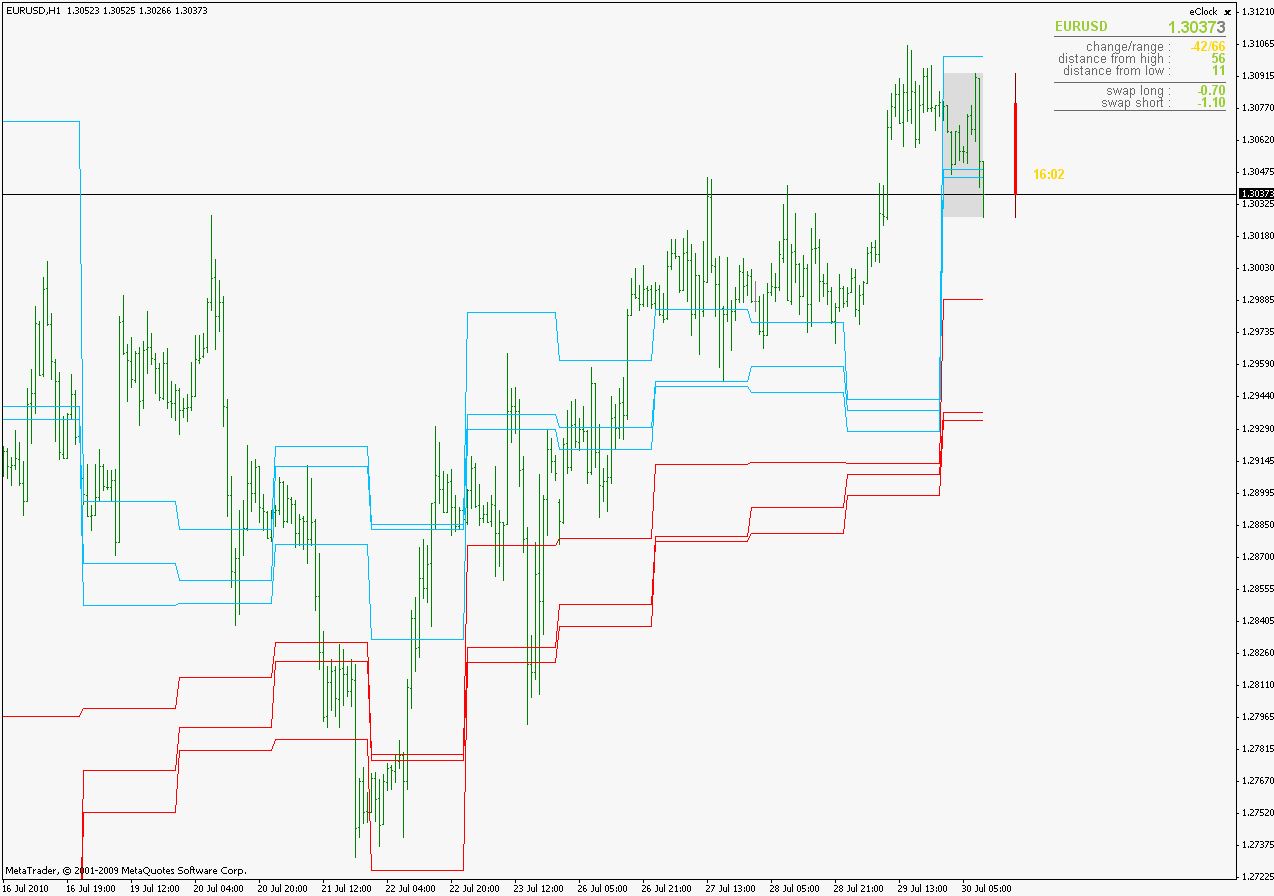In the formula you supplied I used
previous period balance point highest for high

previous period balance point lowest for low

previous period last balance point (a sort of close) for balance point
regards

Fudomyo:
Wow! That was amazingly fast. Thank you so much.

That was a great idea to add the flexibility of adjusting the dbpLength and target time frame. very nice.Is there a way to have the indicator draw the balance point as a horizontal line and factor support and resistance levels off of it based on these calculations?

Resistance1 = 2 * BalancePoint - low;

Resistance2 = BalancePoint + (high - low);

Resistance3 = high + 2 * (BalancePoint - low);

Support1 = 2 * BalancePoint - high;

Support2 = BalancePoint - (high - low);

Support3 = low - 2 * (high - BalancePoint);
Files: